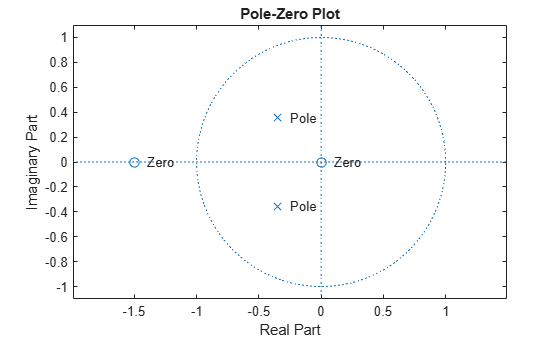tf2zp

Convert transfer function filter parameters to zero-pole-gain form

Description

example

[z,p,k] = tf2zp(b,a) finds the matrix of zeros z, the vector of poles p, and the associated vector of gains k from the transfer function parameters b and a. The function converts a polynomial transfer-function representation

$H\left(s\right)=\frac{B\left(s\right)}{A\left(s\right)}=\frac{{b}_{1}{s}^{n-1}+\cdots +{b}_{n-1}s+{b}_{n}}{{a}_{1}{s}^{m-1}+\cdots +{a}_{m-1}s+{a}_{m}}$

of a single-input/multi-output (SIMO) continuous-time system to a factored transfer function form

$H\left(s\right)=\frac{Z\left(s\right)}{P\left(s\right)}=k\frac{\left(s-{z}_{1}\right)\left(s-{z}_{2}\right)\cdots \left(s-{z}_{m}\right)}{\left(s-{p}_{1}\right)\left(s-{p}_{2}\right)\cdots \left(s-{p}_{n}\right)}.$

Note

Use tf2zp when working with positive powers (s2 + s + 1), such as in continuous-time transfer functions. A similar function, tf2zpk, is more useful when working with transfer functions expressed in inverse powers (1 + z–1 + z–2).

Examples

collapse all

Generate a system with the following transfer function.

$H\left(s\right)=\frac{2{s}^{2}+3s}{{s}^{2}+\frac{1}{\sqrt{2}}s+\frac{1}{4}}=\frac{2\phantom{\rule{0.16666666666666666em}{0ex}}\left(s-0\right)\phantom{\rule{0.16666666666666666em}{0ex}}\left(s-\left(-\frac{3}{2}\right)\right)}{\left(s-\frac{-1}{2\sqrt{2}}\left(1-j\right)\right)\phantom{\rule{0.16666666666666666em}{0ex}}\left(s-\frac{-1}{2\sqrt{2}}\left(1+j\right)\right)}$

Find the zeros, poles, and gain of the system. Use eqtflength to ensure the numerator and denominator have the same length.

b = [2 3];
a = [1 1/sqrt(2) 1/4];

[b,a] = eqtflength(b,a);
[z,p,k] = tf2zp(b,a)
z = 2×1

0
-1.5000

p = 2×1 complex

-0.3536 + 0.3536i
-0.3536 - 0.3536i

k = 2

Plot the poles and zeros to verify that they are in the expected locations.

fvtool(b,a,'polezero')
text(real(z)+.1,imag(z),'Zero')
text(real(p)+.1,imag(p),'Pole')Input Arguments

collapse all

Transfer function numerator coefficients, specified as a vector or matrix. If b is a matrix, then each row of b corresponds to an output of the system. b contains the coefficients in descending powers of s. The number of columns of b must be less than or equal to the length of a.

Data Types: single | double

Transfer function denominator coefficients, specified as a vector. a contains the coefficients in descending powers of s.

Data Types: single | double

Output Arguments

collapse all

Zeros of the system, returned as a matrix. z contains the numerator zeros in its columns. z has as many columns as there are outputs.

Poles of the system, returned as a column vector. p contains the pole locations of the denominator coefficients of the transfer function.

Gains of the system, returned as a column vector. k contains the gains for each numerator transfer function.